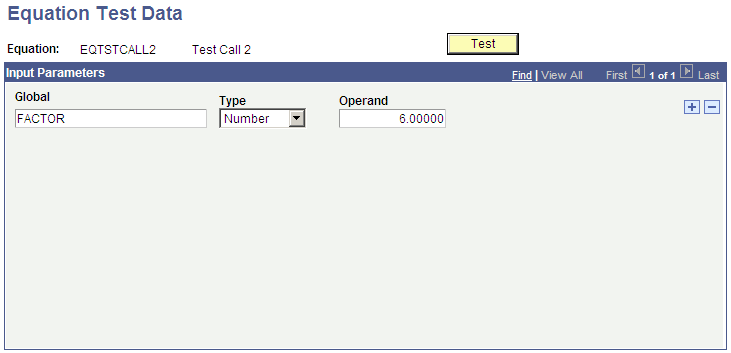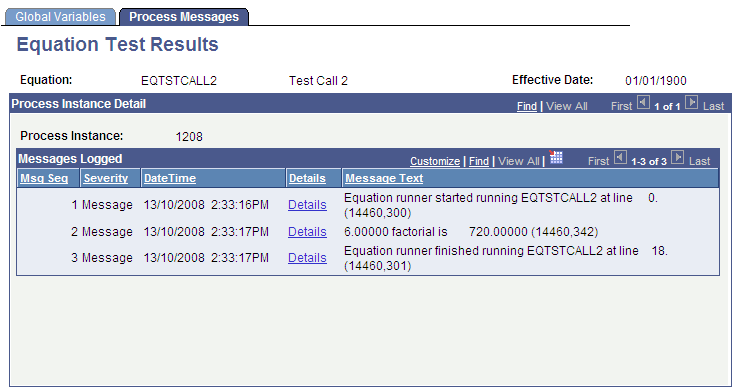Testing Equations

This section discusses how to:

• Define equation test data.

• View equation test run details.

To test the equation, you may need to set up test data. In an equation for which the sole function is to add a few numbers together, no test data is needed because all the data elements are provided. However, to test an equation that references keys in the table or passes global variables, the equation must have test data present.

Page Name

Definition Name

Usage

Equation Test Data

EQTN_TEST_DATA

select Set Up SACR, then select Common Definitions, then select Equation Engine, then select Equation Test Data, then select Equation Test Data

Define equation test data.

Equation Test Results - Process Messages

EQTN_PRCS_MSGS

Click the Test button on the Equation Test Data page.

View the equation test run details.

Access the Equation Test Data page (select Set Up SACR, then select Common Definitions, then select Equation Engine, then select Equation Test Data).

Image: Equation Test Data page

This example illustrates the fields and controls on the Equation Test Data page. You can find definitions for the fields and controls later on this page.Use this page to define the name of the global variable, type, and operand.

After you have defined all the necessary variables and entered data on the Equation Test Data page, click the Test button.

The system displays the Equation Test Results - Process Messages page.

Note: You can also run this process as a batch process rather than initiating it by the Test button.

To test the equation in batch, select the Run Equation option select (Set Up SACR, then select Common Definitions, then select Equation Engine, then select Run Equation, then select Run an Equation).

You can also print the equation that has been established in batch by selecting the Print Equation option select (Set Up SACR, then select Common Definitions, then select Equation Engine, then select Print Equations, then select Print an Equation).

Access the Equation Test Results page: Process Messages tab (click the Test button on the Equation Test Data page).

Image: Equation Test Results page: Process Messages tab

This example illustrates the fields and controls on the Equation Test Results page: Process Messages tab. You can find definitions for the fields and controls later on this page.The page displays the messages associated with the equation test run.

Select the Global Variables tab.

Image: Equation Test Results page: Global Variables tab

This example illustrates the fields and controls on the Equation Test Results page: Global Variables tab. You can find definitions for the fields and controls later on this page.The Global Variable page displays the global variables used within the current equation run.